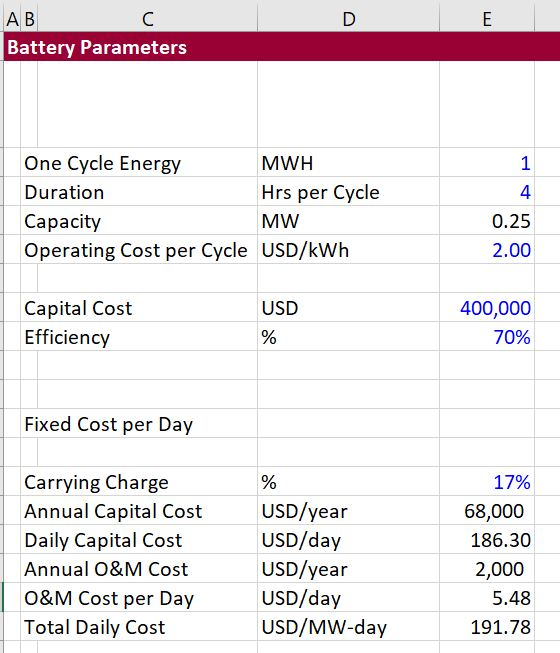# Battery Analysis and Merchant Prices

This page evaluates the economics of battery storage in the context of merchant prices.  Battery storage is used to purchase electricity at low prices; store the electricity and then re-sell the electricity at higher prices.

I received an e-mail that included the following information.  This e-mail illustrates both how to make a simple evaluation of a battery and also the distortions in the analysis.  It may be a good introduction to evaluation of batteries.

• The cost of an installed 1 MWh battery in Canada is roughly \$1M
• Assuming a 10% interest rate, the daily carrying cost of that is \$1M*10%/365 = \$274
• Assuming one battery cycle per day, the daily revenue the battery would have to generate is \$274
• Assuming an 80% efficiency factor, this grosses up to \$342
• This is the intraday price arbitrage that would be needed; \$342. IE, sell power at \$342/MWh higher than you buy it for at another time in the day.

The problems with this analysis include:

1. The carrying charge can be very different than a simple discount rate.  This is particularly true when the lifetime is short or when there is degradation in the output from a battery.
2. The amount of money requried to generate the income is computed correctly, this can be evaluated by examining the on-peak versus off-peak power as shown below.
3. The cost of batteries has come down significantly from the USD 1000/kWh shown above.
4. The ancillary services may add a lot to the value of a battery.

## Simple Analysis of Battery

You can construct a simple analysis of a battery using carrying charge rates, the cost per capacity or per cycle of energy and the cost of O&M just like for other technologies.  Then you can compare the cost of the battery on a daily basis with merchant prices.  You can do this for a single day or over the course of a year.

The first step is to understand the fixed costs of a battery which can be converted first to the cost per year and then the cost per day.  In the example below I have used data from Lazard which quotes the cost per kWh.  I have assumed a cost per one cycle of storage per kWh of USD400/kWh.  For a battery with a duration of 4 hours, the cost per kW is USD 1,000/kW.A big question in the analysis is the annual carrying charge.  You can look at the pages that work through carrying charge to understand this number.  Once the daily cost is computed you can test whether it is possible to achive this value from the difference between off-peak and on-peak prices.  To illustrate this, consider the following prices over the course of a day and the value of storing energy and then releasing that energy with a loss.The final part of the analysis involves comparing the net benefits with the costs.  Using the example above, the net benefits of the battery are less than the cost of the battery as shown below.## Video on Merchant Prices and Use of Batteries for Arbitrage

The video below illustrates how to use merchant prices in evaluating the economics of batteries and storage.